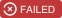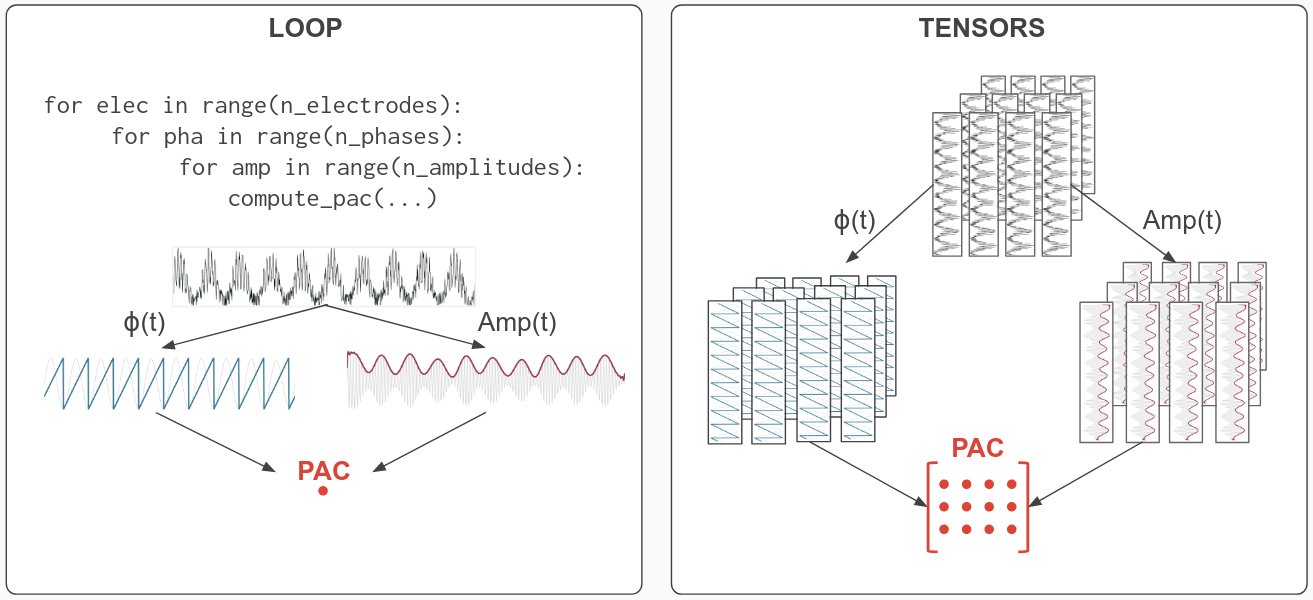# Tensorpac¶

Tensorpac is an open-source Python toolbox for computing Phase-Amplitude Coupling (PAC) using tensors and parallel computing. This software provides a modular implementation which allows one to combine existing methods for measuring PAC and chance distribution.## Tensor based implementation¶

In general, most of the softwares implemented the PAC in a vectorial fashion. This means that the PAC is computed between a single vector of phase and a single vector of amplitude. One of the limitation of this approach is that it can be relatively slow when exploring multi-dimensional data (e.g number of electrodes / sensors, number of trials, several frequency bands etc.). Tensorpac uses a different approach, using the Einstein summation, where the PAC is implemented in order to support multi-dimensional arrays (i.e tensors). This type of implementation can drastically decrease computational cost, especially if it’s combined with parallel computing as it is the case in Tensorpac.On the left, a traditional loop implementation to compute PAC between vectors. On the right, an illustration of the tensor-based implementation.